# Applications of differential subordinations for certain classes of p-valent functions associated with generalized Srivastava-Attiya operator

## Abstract

The object of the present paper is to investigate some inclusion relations and other interesting properties for certain classes of p-valent functions involving generalized Srivastava-Attiya operator by using the principle of differential subordination.

MSC:30C45.

## 1 Introduction

Let $A\left(p\right)$ be the class of functions which are analytic and p-valent in the unit disc $U=\left\{z\in \mathbb{C}:|z|<1\right\}$ of the form

$f\left(z\right)={z}^{p}+\sum _{k=1}^{\mathrm{\infty }}{a}_{k+p}{z}^{k+p}\phantom{\rule{1em}{0ex}}\left(p\in \mathbb{N}=\left\{1,2,\dots \right\}\right).$
(1.1)

Let also $A\left(1\right)={A}_{1}$. For $g\left(z\right)\in A\left(p\right)$, given by $g\left(z\right)={z}^{p}+{\sum }_{k=1}^{\mathrm{\infty }}{b}_{k+p}{z}^{k+p}$, the Hadamard product (or convolution) of $f\left(z\right)$ and $g\left(z\right)$ is defined by

$\left(f\ast g\right)\left(z\right)={z}^{p}+\sum _{k=1}^{\mathrm{\infty }}{a}_{k+p}{b}_{k+p}{z}^{k+p}=\left(g\ast f\right)\left(z\right).$
(1.2)

Next, in the usual notation, let $\mathrm{\Phi }\left(z,s,a\right)$ denote the Hurwitz-Lerch Zeta function defined as follows:(1.3)

For further interesting properties and characteristics of the Hurwitz-Lerch Zeta function $\mathrm{\Phi }\left(z,s,a\right)$ see [2, 5, 8, 9, 11], and .

Recently, Srivastava and Attiya  have introduced the linear operator ${L}_{s,b}:{A}_{1}\to {A}_{1}$, defined in terms of the Hadamard product by

${L}_{s,b}\left(f\right)\left(z\right)={G}_{s,b}\left(z\right)\ast f\left(z\right)\phantom{\rule{1em}{0ex}}\left(z\in U;b\in \mathbb{C}\mathrm{\setminus }{\mathbb{Z}}_{0}^{-};s\in \mathbb{C}\right),$
(1.4)

where

${G}_{s,b}={\left(1+b\right)}^{s}\left[\mathrm{\Phi }\left(z,s,b\right)-{b}^{-s}\right]\phantom{\rule{1em}{0ex}}\left(z\in U\right).$
(1.5)

The Srivastava-Attiya operator ${L}_{s,b}$ contains, among its special cases, the integral operators introduced and investigated by Alexander , Libera  and Jung et al. .

Analogous to ${L}_{s,b}$, Liu  defined the operator ${J}_{p,s,b}:A\left(p\right)\to A\left(p\right)$ by

${J}_{p,s,b}\left(f\right)\left(z\right)={G}_{p,s,b}\left(z\right)\ast f\left(z\right)\phantom{\rule{1em}{0ex}}\left(z\in U;b\in \mathbb{C}\mathrm{\setminus }{\mathbb{Z}}_{0}^{-};s\in \mathbb{C};p\in \mathbb{N}\right),$
(1.6)

where

${G}_{p,s,b}={\left(1+b\right)}^{s}\left[{\mathrm{\Phi }}_{p}\left(z,s,b\right)-{b}^{-s}\right]$

and

${\mathrm{\Phi }}_{p}\left(z,s,b\right)=\frac{1}{{b}^{s}}+\sum _{k=0}^{\mathrm{\infty }}\frac{{z}^{k+p}}{{\left(k+1+b\right)}^{s}}.$
(1.7)

It is easy to observe from (1.6) and (1.7) that

${J}_{p,s,b}\left(f\right)\left(z\right)={z}^{p}+\sum _{k=1}^{\mathrm{\infty }}{\left(\frac{1+b}{k+1+b}\right)}^{s}{a}_{k+p}{z}^{k+p}.$
(1.8)

We note that

1. (i)

${J}_{p,0,b}\left(f\right)\left(z\right)=f\left(z\right)$;

2. (ii)

${J}_{1,1,0}\left(f\right)\left(z\right)=Lf\left(z\right)={\int }_{0}^{z}\frac{f\left(t\right)}{t}\phantom{\rule{0.2em}{0ex}}dt$ ($f\in {A}_{1}$), where the operator L was introduced by Alexander ;

3. (iii)

${J}_{1,s,b}\left(f\right)\left(z\right)={L}_{s,b}f\left(z\right)$ ($s\in \mathbb{C}$$b\in \mathbb{C}\mathrm{\setminus }{\mathbb{Z}}_{0}^{-}$), where the operator ${L}_{s,b}$ was introduced by Srivastava-Attiya ;

4. (iv)

${J}_{p,1,\mu +p-1}\left(f\right)\left(z\right)={F}_{\mu ,p}\left(f\right)\left(z\right)$ ($\mu >-p$$p\in \mathbb{N}$), where the operator ${F}_{\mu ,p}$ was introduced by Choi et al. ;

5. (v)

${J}_{p,\alpha ,p}\left(f\right)\left(z\right)={I}_{p}^{\alpha }f\left(z\right)$ ($\alpha >0$$p\in \mathbb{N}$), where the operator ${I}_{p}^{\alpha }$ was introduced by Shams et al. ;

6. (vi)

${J}_{p,\gamma ,p-1}\left(f\right)\left(z\right)={J}_{p}^{\gamma }f\left(z\right)$ ($\gamma \in {\mathbb{N}}_{0}=\mathbb{N}\cup \left\{0\right\}$$p\in \mathbb{N}$), where the operator ${J}_{p}^{\gamma }$ was introduced by El-Ashwah and Aouf ;

7. (vii)

${J}_{p,\gamma ,p+l-1}\left(f\right)\left(z\right)={J}_{p}^{\gamma }\left(l\right)f\left(z\right)$ ($\gamma \in {\mathbb{N}}_{0}$$p\in \mathbb{N}$$l\ge 0$), where the operator ${J}_{p}^{\gamma }\left(l\right)$ was introduced by El-Ashwah and Aouf .

It follows from (1.8) that

$z{\left({J}_{p,s,b}\left(f\right)\left(z\right)\right)}^{\mathrm{\prime }}=\left(b+1\right){J}_{p,s-1,b}\left(f\right)\left(z\right)-\left(b+1-p\right){J}_{p,s,b}\left(f\right)\left(z\right).$
(1.9)

For two analytic functions $f,g\in A\left(p\right)$, we say that f is subordinate to g, written $f\left(z\right)\prec g\left(z\right)$ if there exists a Schwarz function $w\left(z\right)$, which (by definition) is analytic in U with $w\left(0\right)=0$ and $|w\left(z\right)|<1$ for all $z\in U$, such that $f\left(z\right)=g\left(w\left(z\right)\right)$$z\in U$. Furthermore, if the function $g\left(z\right)$ is univalent in U, then we have the following equivalence (see ):

$f\left(z\right)\prec g\left(z\right)\phantom{\rule{1em}{0ex}}⇔\phantom{\rule{1em}{0ex}}f\left(0\right)=g\left(0\right)\phantom{\rule{1em}{0ex}}\text{and}\phantom{\rule{1em}{0ex}}f\left(U\right)\subset g\left(U\right).$

Definition 1 For fixed parameters A and B, with $-1\le B, we say that $f\in A\left(p\right)$ is in the class ${S}_{p}^{s,b}\left(A,B\right)$ if it satisfies the following subordination condition:

$\frac{{\left({J}_{p,s,b}\left(f\right)\left(z\right)\right)}^{\mathrm{\prime }}}{p{z}^{p-1}}\prec \frac{1+Az}{1+Bz}\phantom{\rule{1em}{0ex}}\left(p\in \mathbb{N}\right).$
(1.10)

In view of the definition of subordination (1.10) is equivalent to the following condition:

$|\frac{\frac{{\left({J}_{p,s,b}\left(f\right)\left(z\right)\right)}^{\mathrm{\prime }}}{p{z}^{p-1}}-1}{B\frac{{\left({J}_{p,s,b}\left(f\right)\left(z\right)\right)}^{\mathrm{\prime }}}{p{z}^{p-1}}-A}|<1\phantom{\rule{1em}{0ex}}\left(z\in U\right).$

For convenience, we write ${S}_{p}^{s,b}\left(1-\frac{2\eta }{p},-1\right)={S}_{p}^{s,b}\left(\eta \right)$, where ${S}_{p}^{s,b}\left(\eta \right)$ denotes the class of functions in $A\left(p\right)$ satisfying the inequality

$Re\left(\frac{{\left({J}_{p,s,b}\left(f\right)\left(z\right)\right)}^{\mathrm{\prime }}}{p{z}^{p-1}}\right)>\eta \phantom{\rule{1em}{0ex}}\left(0\le \eta <1;p\in \mathbb{N};z\in U\right).$

In the present paper, we investigate some inclusion relations and other interesting properties for certain classes of p-valent functions involving an integral operator.

## 2 Preliminaries

To establish our main results, we need the following lemmas.

Lemma 1 ([13, 14])

Let h be analytic and convex (univalent) in U with$h\left(0\right)=1$. Suppose also that the function φ given by

$\phi \left(z\right)=1+{c}_{m}{z}^{m}+{c}_{m+1}{z}^{m+1}+\cdots ,$
(2.1)

is analytic in U, where m is a positive integer. If

$\phi \left(z\right)+\frac{z{\phi }^{\mathrm{\prime }}\left(z\right)}{\varrho }\prec h\left(z\right)\phantom{\rule{1em}{0ex}}\left(Re\left\{\varrho \right\}\ge 0;\varrho \ne 0\right),$
(2.2)

then

$\phi \left(z\right)\prec \psi \left(z\right)=\frac{\varrho }{m}{z}^{-\frac{\varrho }{m}}{\int }_{0}^{z}{t}^{\frac{\varrho }{m}-1}h\left(t\right)\phantom{\rule{0.2em}{0ex}}dt\prec h\left(z\right)$
(2.3)

and$\psi \left(z\right)$is the best dominant of (2.2).

We denote by $H\left(\varrho \right)$ the class of functions $\mathrm{\Phi }\left(z\right)$ given by

$\mathrm{\Phi }\left(z\right)=1+{c}_{1}z+{c}_{2}{z}^{2}+\cdots ,$
(2.4)

which are analytic in U and satisfy the following inequality:

$Re\left\{\mathrm{\Phi }\left(z\right)\right\}>\varrho \phantom{\rule{1em}{0ex}}\left(0\le \varrho <1;z\in U\right).$

Lemma 2 ()

Let the function$\mathrm{\Phi }\left(z\right)\in H\left(\varrho \right)$, where$\mathrm{\Phi }\left(z\right)$given by (2.4). Then

$Re\left\{\mathrm{\Phi }\left(\varrho \right)\right\}\ge 2\varrho -1+\frac{2\left(1-\varrho \right)}{1+|z|}\phantom{\rule{1em}{0ex}}\left(0\le \varrho <1;z\in U\right).$

Lemma 3 ()

For$0\le {\varrho }_{1}$, ${\varrho }_{2}<1$,

$H\left({\varrho }_{1}\right)\ast H\left({\varrho }_{2}\right)\subset H\left({\varrho }_{3}\right),\phantom{\rule{1em}{0ex}}{\varrho }_{3}=1-2\left(1-{\varrho }_{1}\right)\left(1-{\varrho }_{2}\right).$

The result is best possible.

Lemma 4 ()

Let μ be a positive measure on the unit interval$\left[0,1\right]$. Let$g\left(z,t\right)$be a complex valued function defined on$U×\left[0,1\right]$such that$g\left(0,t\right)$is analytic in U for each$t\in \left[0,1\right]$and such that$g\left(z,0\right)$is μ integrable on$\left[0,1\right]$for all$z\in U$. In addition, suppose that$Re\left\{g\left(z,t\right)\right\}>0$, $g\left(-r,t\right)$is real and

$Re\left\{\frac{1}{g\left(z,t\right)}\right\}\ge \frac{1}{g\left(-r,t\right)}\phantom{\rule{1em}{0ex}}\left(|z|\le r<1;t\in \left[0,1\right]\right).$

If G is defined by

$G\left(z\right)={\int }_{0}^{1}g\left(z,t\right)\phantom{\rule{0.2em}{0ex}}d\mu \left(t\right),$

then

$Re\left\{\frac{1}{G\left(z\right)}\right\}\ge \frac{1}{G\left(-r\right)}\phantom{\rule{1em}{0ex}}\left(|z|\le r<1\right).$

Lemma 5 ()

Let the function g be analytic in U with$g\left(0\right)=1$and$Re\left\{g\left(z\right)\right\}>\frac{1}{2}$ ($z\in U$). Then, for any function F analytic in U, $\left(g\ast F\right)\left(U\right)$is contained in the convex hull of$F\left(U\right)$.

Lemma 6 ()

Let φ be analytic in U with$\phi \left(0\right)=1$and$\phi \left(z\right)=0$for$0<|z|<1$and let$A,B\in \mathbb{C}$with$A\ne B$, $|B|\le 1$.

1. (i)

Let$B\ne 0$and$\gamma \in {\mathbb{C}}^{\ast }=\mathbb{C}\mathrm{\setminus }\left\{0\right\}$satisfy either$|\frac{\gamma \left(A-B\right)}{B}-1|\le 1$or$|\frac{\gamma \left(A-B\right)}{B}+1|\le 1$. If φ satisfies

$1+\frac{z{\phi }^{\mathrm{\prime }}\left(z\right)}{\gamma \phi \left(z\right)}\prec \frac{1+Az}{1+Bz},$
(2.5)

then

$\phi \left(z\right)\prec {\left(1+Bz\right)}^{\gamma \left(\frac{A-B}{B}\right)},$

and this is best dominant.

1. (ii)

Let$B=0$and$\gamma \in {\mathbb{C}}^{\ast }$be such that$|\gamma A|<\pi$. If φ satisfies (2.5), then

$\phi \left(z\right)\prec {e}^{\gamma Az}$

and this is the best dominant.

For real or complex numbers a n and c ($c\notin {\mathbb{Z}}_{0}^{-}$) and $z\in U$, the Gaussian hypergeometric function defined by

$\begin{array}{rl}{}_{2}F_{1}\left(a,n;c;z\right)& =1+\frac{an}{c}\cdot \frac{z}{1!}+\frac{a\left(a+1\right)n\left(n+1\right)}{c\left(c+1\right)}\cdot \frac{{z}^{2}}{2!}+\cdots \\ =\sum _{k=0}^{\mathrm{\infty }}\frac{{\left(a\right)}_{k}{\left(n\right)}_{k}}{{\left(c\right)}_{k}}\frac{{z}^{k}}{k!},\end{array}$
(2.6)

where ${\left(d\right)}_{k}=d\left(d+1\right)\cdots \left(d+k-1\right)$ and ${\left(d\right)}_{0}=1$. We note that the series defined by (2.6) converges absolutely for $z\in U$, and hence, ${}_{2}F_{1}$ represents an analytic function in U (see, for details, , Ch.14]).

Lemma 7 ()

For real or complex numbers a, n and c ($c\notin {\mathbb{Z}}_{0}^{-}$)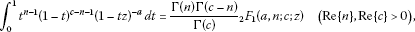(2.7)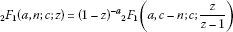(2.8)

and

${}_{2}F_{1}\left(a,n;c;z\right){=}_{2}{F}_{1}\left(n,a;c;z\right).$
(2.9)

## 3 Main results

Unless otherwise mentioned, we assume throughout this paper that $-1\le B, $s\in \mathbb{C}$, $b\in \mathbb{C}\mathrm{\setminus }{\mathbb{Z}}_{0}^{-}$, $p\in \mathbb{N}\mathrm{\setminus }\left\{1\right\}$, $0<\alpha \le 1$, m is a positive integer and the powers are understood as principle values.

Theorem 1 Let f given by (1.1) satisfy the following subordination condition:

$\left(1-\alpha \right)\frac{{\left({J}_{p,s,b}\left(f\right)\left(z\right)\right)}^{\mathrm{\prime }}}{p{z}^{p-1}}+\alpha \frac{{\left({J}_{p,s,b}\left(f\right)\left(z\right)\right)}^{\mathrm{\prime }\mathrm{\prime }}}{p\left(p-1\right){z}^{p-2}}\prec \frac{1+Az}{1+Bz}.$
(3.1)

Then

$\frac{{\left({J}_{p,s,b}\left(f\right)\left(z\right)\right)}^{\mathrm{\prime }}}{p{z}^{p-1}}\prec \mathrm{\Psi }\left(z\right)\prec \frac{1+Az}{1+Bz},$
(3.2)

where

$\mathrm{\Psi }\left(z\right)=\left\{\begin{array}{cc}\frac{A}{B}+\left(1-\frac{A}{B}\right){{\left(1+Bz\right)}^{-1}}_{2}{F}_{1}\left(1,1;\frac{p-1}{m\alpha }+1;\frac{Bz}{1+Bz}\right)\hfill & \text{for}B\ne 0,\hfill \\ 1+\frac{p-1}{m\alpha +p-1}Az\hfill & \text{for}B=0,\hfill \end{array}$
(3.3)

is the best dominant of (3.2). Furthermore,

$f\in {S}_{p}^{s,b}\left(\beta \right),$
(3.4)

where

$\beta =\left\{\begin{array}{cc}\frac{A}{B}+\left(1-\frac{A}{B}\right)\left(1-B\right)^{-1}{}_{2}{F}_{1}\left(1,1;\frac{p-1}{m\alpha }+1;\frac{B}{B-1}\right)\hfill & \text{for}B\ne 0,\hfill \\ 1-\frac{p-1}{m\alpha +p-1}A\hfill & \text{for}B=0.\hfill \end{array}$
(3.5)

The estimate (3.4) is best possible.

Proof Let

$\theta \left(z\right)=\frac{{\left({J}_{p,s,b}\left(f\right)\left(z\right)\right)}^{\mathrm{\prime }}}{p{z}^{p-1}}\phantom{\rule{1em}{0ex}}\left(z\in U\right),$
(3.6)

where θ is of the form (2.1) and is analytic in U. Differentiating (3.6) with respect to z, we get

$\left(1-\alpha \right)\frac{{\left({J}_{p,s,b}\left(f\right)\left(z\right)\right)}^{\mathrm{\prime }}}{p{z}^{p-1}}+\alpha \frac{{\left({J}_{p,s,b}\left(f\right)\left(z\right)\right)}^{\mathrm{\prime }\mathrm{\prime }}}{p\left(p-1\right){z}^{p-2}}=\theta \left(z\right)+\frac{\alpha }{p-1}z{\theta }^{\mathrm{\prime }}\left(z\right)\prec \frac{1+Az}{1+Bz}.$

Applying Lemma 1 for $\varrho =\frac{p-1}{\alpha }$ and Lemma 7, we have

$\begin{array}{rcl}\frac{{\left({J}_{p,s,b}\left(f\right)\left(z\right)\right)}^{\mathrm{\prime }}}{p{z}^{p-1}}& \prec & \mathrm{\Psi }\left(z\right)=\frac{p-1}{m\alpha }{z}^{-\frac{p-1}{m\alpha }}{\int }_{0}^{z}{t}^{\frac{p-1}{m\alpha }-1}\left(\frac{1+At}{1+Bt}\right)\phantom{\rule{0.2em}{0ex}}dt\\ =& \left\{\begin{array}{cc}\frac{A}{B}+\left(1-\frac{A}{B}\right)\left(1+Bz\right)^{-1}{}_{2}{F}_{1}\left(1,1;\frac{p-1}{m\alpha }+1;\frac{Bz}{1+Bz}\right)\hfill & \text{for}B\ne 0,\hfill \\ 1+\frac{p-1}{m\alpha +p-1}Az\hfill & \text{for}B=0.\hfill \end{array}\end{array}$

This proves the assertion (3.2) of Theorem 1. Next, in order to prove the assertion (3.4) of Theorem 1, it suffices to show that

$\underset{|z|<1}{inf}\left\{Re\left(\mathrm{\Psi }\left(z\right)\right)\right\}=\mathrm{\Psi }\left(-1\right).$

Indeed, we have

$Re\left\{\frac{1+Az}{1+Bz}\right\}\ge \frac{1-Ar}{1-Br}\phantom{\rule{1em}{0ex}}\left(|z|\le r<1\right).$

Setting

$G\left(z,\zeta \right)=\frac{1+A\zeta z}{1+B\zeta z}\phantom{\rule{1em}{0ex}}\text{and}\phantom{\rule{1em}{0ex}}d\nu \left(\zeta \right)=\frac{p-1}{m\alpha }{\zeta }^{\frac{p-1}{m\alpha }-1}\phantom{\rule{0.2em}{0ex}}d\zeta \phantom{\rule{1em}{0ex}}\left(0\le \zeta \le 1\right),$

which is a positive measure on the closed interval $\left[0,1\right]$, we get

$\mathrm{\Psi }\left(z\right)={\int }_{0}^{1}G\left(z,\zeta \right)\phantom{\rule{0.2em}{0ex}}d\nu \left(\zeta \right).$

Then

$Re\left\{\mathrm{\Psi }\left(z\right)\right\}\ge {\int }_{0}^{1}\frac{1-A\zeta r}{1-B\zeta r}\phantom{\rule{0.2em}{0ex}}d\nu \left(\zeta \right)=\mathrm{\Psi }\left(-r\right)\phantom{\rule{1em}{0ex}}\left(|z|\le r<1\right).$

Letting $r\to {1}^{-}$ in the above inequality, we obtain the assertion (3.4). Finally, the estimate (3.4) is best possible as Ψ is the best dominant of (3.2). This completes the proof of Theorem 1. □

Theorem 2 If$f\in {S}_{p}^{s,b}\left(\eta \right)$ ($0\le \eta <1$), then

$Re\left\{\left(1-\alpha \right)\frac{{\left({J}_{p,s,b}\left(f\right)\left(z\right)\right)}^{\mathrm{\prime }}}{p{z}^{p-1}}+\alpha \frac{{\left({J}_{p,s,b}\left(f\right)\left(z\right)\right)}^{\mathrm{\prime }\mathrm{\prime }}}{p\left(p-1\right){z}^{p-2}}\right\}>\eta \phantom{\rule{1em}{0ex}}\left(|z|

where

$R={\left\{\frac{\sqrt{{\left(p-1\right)}^{2}+{\left(m\alpha \right)}^{2}}-m\alpha }{p-1}\right\}}^{\frac{1}{m}}.$
(3.7)

The result is best possible.

Proof Let $f\in {S}_{p}^{s,b}\left(\eta \right)$, then we write

$\frac{{\left({J}_{p,s,b}\left(f\right)\left(z\right)\right)}^{\mathrm{\prime }}}{p{z}^{p-1}}=\eta +\left(1-\eta \right)u\left(z\right)\phantom{\rule{1em}{0ex}}\left(z\in U\right),$
(3.8)

where u is of the form (2.1), is analytic in U and has a positive real part in U. Differentiating (3.8) with respect to z, we have

$\frac{1}{1-\eta }\left\{\left(1-\alpha \right)\frac{{\left({J}_{p,s,b}\left(f\right)\left(z\right)\right)}^{\mathrm{\prime }}}{p{z}^{p-1}}+\alpha \frac{{\left({J}_{p,s,b}\left(f\right)\left(z\right)\right)}^{\mathrm{\prime }\mathrm{\prime }}}{p\left(p-1\right){z}^{p-2}}-\eta \right\}=u\left(z\right)+\frac{\alpha }{p-1}z{u}^{\mathrm{\prime }}\left(z\right).$
(3.9)

Applying the following well-known estimate :

$\frac{|z{u}^{\mathrm{\prime }}\left(z\right)|}{Re\left\{u\left(z\right)\right\}}\le \frac{2m{r}^{m}}{1-{r}^{2m}}\phantom{\rule{1em}{0ex}}\left(|z|=r<1\right),$

in (3.9), we have(3.10)

such that the right-hand side of (3.10) is positive, if $r, where R is given by (3.7).

In order to show that the bound R is best possible, we consider the function $f\in A\left(p\right)$ defined by

$\frac{{\left({J}_{p,s,b}\left(f\right)\left(z\right)\right)}^{\mathrm{\prime }}}{p{z}^{p-1}}=\eta +\left(1-\eta \right)\frac{1+{z}^{m}}{1-{z}^{m}}\phantom{\rule{1em}{0ex}}\left(0\le \eta <1;z\in U\right).$

Note that

$\frac{1}{1-\eta }\left\{\left(1-\alpha \right)\frac{{\left({J}_{p,s,b}\left(f\right)\left(z\right)\right)}^{\mathrm{\prime }}}{p{z}^{p-1}}+\alpha \frac{{\left({J}_{p,s,b}\left(f\right)\left(z\right)\right)}^{\mathrm{\prime }\mathrm{\prime }}}{p\left(p-1\right){z}^{p-2}}-\eta \right\}=\frac{\left(p-1\right)\left(1-{z}^{2m}\right)-2\alpha m{z}^{m}}{\left(p-1\right){\left(1-{z}^{m}\right)}^{2}}=0,$

for $z={R}^{\cdot }exp\left\{\frac{\mathbf{i}\mathbit{\pi }}{\mathbf{m}}\right\}$. This completes the proof of Theorem 2. □

For a function $f\in A\left(p\right)$, the generalized Bernardi-Libera-Livingston integral operator ${F}_{\mu ,p}$ is defined by

$\begin{array}{rl}{F}_{\mu ,p}\left(f\right)\left(z\right)& =\frac{\mu +p}{{z}^{\mu }}{\int }_{0}^{z}{t}^{\mu -1}f\left(t\right)\phantom{\rule{0.2em}{0ex}}dt=\left({z}^{p}+\sum _{k=1}^{\mathrm{\infty }}\frac{\mu +p}{\mu +p+k}{z}^{k+p}\right)\ast f\left(z\right)\\ =z^{p}{}_{2}{F}_{1}\left(1,\mu +p;\mu +p+1;z\right)\ast f\left(z\right)\phantom{\rule{1em}{0ex}}\left(\mu >-p;z\in U\right).\end{array}$
(3.11)

From (1.8) and (3.11), we have

$z{\left({J}_{p,s,b}{\mathrm{F}}_{\mu ,p}\left(f\left(z\right)\right)\right)}^{\mathrm{\prime }}=\left(\mu +p\right){J}_{p,s,b}\left(f\right)\left(z\right)-\mu {J}_{p,s,b}{\mathrm{F}}_{\mu ,p}\left(f\left(z\right)\right)\phantom{\rule{1em}{0ex}}\left(\mu >-p;z\in U\right)$
(3.12)

and

${J}_{p,s,b}{\mathrm{F}}_{\mu ,p}\left(f\left(z\right)\right)={\mathrm{F}}_{\mu ,p}\left({J}_{p,s,b}\left(f\right)\left(z\right)\right).$

Theorem 3 Let$f\in {S}_{p}^{s,b}\left(A,B\right)$and${F}_{\mu ,p}$be defined by (3.11). Then

$\frac{{\left({J}_{p,s,b}{\mathrm{F}}_{\mu ,p}\left(f\left(z\right)\right)\right)}^{\mathrm{\prime }}}{p{z}^{p-1}}\prec \mathrm{\Phi }\left(z\right)\prec \frac{1+Az}{1+Bz},$
(3.13)

where

$\mathrm{\Phi }\left(z\right)=\left\{\begin{array}{cc}\frac{A}{B}+\left(1-\frac{A}{B}\right)\left(1+Bz\right)^{-1}{}_{2}{F}_{1}\left(1,1;\frac{\mu +p}{m}+1;\frac{Bz}{1+Bz}\right)\hfill & \text{for}B\ne 0,\hfill \\ 1+\frac{\mu +p}{\mu +p+m}Az\hfill & \text{for}B=0,\hfill \end{array}$
(3.14)

is the best dominant of (3.13). Furthermore,

$Re\left\{\frac{{\left({J}_{p,s,b}{\mathrm{F}}_{\mu ,p}\left(f\left(z\right)\right)\right)}^{\mathrm{\prime }}}{p{z}^{p-1}}\right\}>\psi \phantom{\rule{1em}{0ex}}\left(z\in U\right),$

where

$\psi =\left\{\begin{array}{cc}\frac{A}{B}+\left(1-\frac{A}{B}\right)\left(1-B\right)^{-1}{}_{2}{F}_{1}\left(1,1;\frac{\mu +p}{m}+1;\frac{B}{B-1}\right)\hfill & \text{for}B\ne 0,\hfill \\ 1-\frac{\mu +p}{\mu +p+m}A\hfill & \text{for}B=0.\hfill \end{array}$

The result is best possible.

Proof Let

$K\left(z\right)=\frac{{\left({J}_{p,s,b}{\mathrm{F}}_{\mu ,p}\left(f\left(z\right)\right)\right)}^{\mathrm{\prime }}}{p{z}^{p-1}}\phantom{\rule{1em}{0ex}}\left(z\in U\right),$
(3.15)

where K is of the form (2.1) and is analytic in U. Using (3.12) in (3.15) and differentiating the resulting equation with respect to z, we have

$\frac{{\left({J}_{p,s,b}\left(f\right)\left(z\right)\right)}^{\mathrm{\prime }}}{p{z}^{p-1}}=K\left(z\right)+\frac{z{K}^{\mathrm{\prime }}\left(z\right)}{p+\mu }\prec \frac{1+Az}{1+Bz}.$

The remaining part of the proof is similar to that of Theorem 1, and so we omit it. □

We note that

$\frac{{\left({J}_{p,s,b}{\mathrm{F}}_{\mu ,p}\left(f\left(z\right)\right)\right)}^{\mathrm{\prime }}}{p{z}^{p-1}}=\frac{p+\mu }{p{z}^{p+\mu }}{\int }_{0}^{z}{t}^{\mu }{\left({J}_{p,s,b}\left(f\right)\left(t\right)\right)}^{\mathrm{\prime }}\phantom{\rule{0.2em}{0ex}}dt\phantom{\rule{1em}{0ex}}\left(f\in A\left(p\right);z\in U\right).$
(3.16)

Putting $A=1-\frac{2\delta }{p}$ ($0\le \delta <1$) and $B=-1$ in Theorem 3 and using (3.16), we obtain the following corollary.

Corollary 1 If$f\in A\left(p\right)$satisfies the following inequality:

$Re\left\{\frac{{\left({J}_{p,s,b}f\left(z\right)\right)}^{\mathrm{\prime }}}{p{z}^{p-1}}\right\}>\delta \phantom{\rule{1em}{0ex}}\left(0\le \delta <1;z\in U\right),$

then

$Re\left\{\frac{p+\mu }{p{z}^{p+\mu }}{\int }_{0}^{z}{t}^{\mu }{\left({J}_{p,s,b}\left(f\right)\left(t\right)\right)}^{\mathrm{\prime }}\phantom{\rule{0.2em}{0ex}}dt\right\}>\frac{\delta }{p}+\left(1-\frac{\delta }{p}\right){\left[}_{2}{F}_{1}\left(1,1;\frac{\mu +p}{m}+1;\frac{1}{2}\right)-1\right]\phantom{\rule{1em}{0ex}}\left(z\in U\right).$

The result is best possible.

Theorem 4 Let$f,g\in A\left(p\right)$satisfy the following inequality:

$Re\left\{\frac{{J}_{p,s,b}\left(g\right)\left(z\right)}{{z}^{p}}\right\}>0\phantom{\rule{1em}{0ex}}\left(z\in U\right).$

If

$|\frac{{J}_{p,s,b}\left(f\right)\left(z\right)}{{J}_{p,s,b}\left(g\right)\left(z\right)}-1|<1\phantom{\rule{1em}{0ex}}\left(z\in U\right),$

then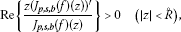where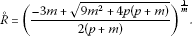(3.17)

Proof Let

$q\left(z\right)=\frac{{J}_{p,s,b}\left(f\right)\left(z\right)}{{J}_{p,s,b}\left(g\right)\left(z\right)}-1={c}_{m}{z}^{m}+{c}_{m+1}{z}^{m+1}+\cdots ,$
(3.18)

where $q\left(z\right)$ is analytic in U with $q\left(0\right)=0$ and $|q\left(z\right)|\le |z{|}^{m}$. Then, by applying the familiar Schwartz Lemma , we have $q\left(z\right)={z}^{m}\mathcal{X}\left(z\right)$, where $\mathcal{X}$ is analytic in U and $|\mathcal{X}\left(z\right)|\le 1$. Therefore (3.18) leads to

${J}_{p,s,b}\left(f\right)\left(z\right)={J}_{p,s,b}\left(g\right)\left(z\right)\left(1+{z}^{m}\mathcal{X}\left(z\right)\right)\phantom{\rule{1em}{0ex}}\left(z\in U\right).$
(3.19)

Differentiating (3.19) logarithmically with respect to z, we have

$\frac{z{\left({J}_{p,s,b}\left(f\right)\left(z\right)\right)}^{\mathrm{\prime }}}{{J}_{p,s,b}\left(f\right)\left(z\right)}=\frac{z{\left({J}_{p,s,b}\left(g\right)\left(z\right)\right)}^{\mathrm{\prime }}}{{J}_{p,s,b}\left(g\right)\left(z\right)}+\frac{{z}^{m}\left\{m\mathcal{X}\left(z\right)+z{\mathcal{X}}^{\mathrm{\prime }}\left(z\right)\right\}}{1+{z}^{m}\mathcal{X}\left(z\right)}.$
(3.20)

Letting

$\omega \left(z\right)=\frac{{J}_{p,s,b}\left(g\right)\left(z\right)}{{z}^{p}}\phantom{\rule{1em}{0ex}}\left(z\in U\right),$

where ω is in the form (2.1), is analytic in U$Re\left\{\omega \left(z\right)\right\}>0$ and

$\frac{z{\left({J}_{p,s,b}\left(g\right)\left(z\right)\right)}^{\mathrm{\prime }}}{{J}_{p,s,b}\left(g\right)\left(z\right)}=\frac{z{\omega }^{\mathrm{\prime }}\left(z\right)}{\omega \left(z\right)}+p,$

then we have

$Re\left\{\frac{z{\left({J}_{p,s,b}\left(f\right)\left(z\right)\right)}^{\mathrm{\prime }}}{{J}_{p,s,b}\left(f\right)\left(z\right)}\right\}\ge p-|\frac{z{\omega }^{\mathrm{\prime }}\left(z\right)}{\omega \left(z\right)}|-|\frac{{z}^{m}\left\{m\mathcal{X}\left(z\right)+z{\mathcal{X}}^{\mathrm{\prime }}\left(z\right)\right\}}{1+{z}^{m}\mathcal{X}\left(z\right)}|.$
(3.21)

$|\frac{{\omega }^{\mathrm{\prime }}\left(z\right)}{\omega \left(z\right)}|\le \frac{2m{r}^{m-1}}{1-{r}^{2m}}\phantom{\rule{1em}{0ex}}\text{and}\phantom{\rule{1em}{0ex}}|\frac{m\mathcal{X}\left(z\right)+z{\mathcal{X}}^{\mathrm{\prime }}\left(z\right)}{1+{z}^{m}\mathcal{X}\left(z\right)}|\le \frac{m}{1-{r}^{m}}\phantom{\rule{1em}{0ex}}\left(|z|=r<1\right),$

in (3.21), we have

$Re\left\{\frac{z{\left({J}_{p,s,b}\left(f\right)\left(z\right)\right)}^{\mathrm{\prime }}}{{J}_{p,s,b}\left(f\right)\left(z\right)}\right\}\ge \frac{p-3m{r}^{m}-\left(p+m\right){r}^{2m}}{1-{r}^{2m}}\phantom{\rule{1em}{0ex}}\left(|z|=r<1\right),$

which is certainly positive, provided that, where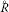is given by (3.17). This completes the proof of Theorem 4. □

Theorem 5 Let$-1\le {B}_{i}<{A}_{i}\le 1$ ($i=1,2$) and$\tau . If each of the functions${f}_{i}\in A\left(p\right)$satisfies the following subordination condition:

$\left(1-\alpha \right)\frac{{\left({J}_{p,s,b}\left({f}_{i}\right)\left(z\right)\right)}^{\mathrm{\prime }}}{p{z}^{p-1}}+\alpha \frac{{\left({J}_{p,s,b}\left({f}_{i}\right)\left(z\right)\right)}^{\mathrm{\prime }\mathrm{\prime }}}{p\left(p-1\right){z}^{p-2}}\prec \frac{1+{A}_{i}z}{1+{B}_{i}z}\phantom{\rule{1em}{0ex}}\left(i=1,2\right),$
(3.22)

then

$\left(1-\alpha \right)\frac{{\left({J}_{p,s,b}\left(F\right)\left(z\right)\right)}^{\mathrm{\prime }}}{p{z}^{p-1}}+\alpha \frac{{\left({J}_{p,s,b}\left(F\right)\left(z\right)\right)}^{\mathrm{\prime }\mathrm{\prime }}}{p\left(p-1\right){z}^{p-2}}\prec \frac{1+\left(1-\frac{2\tau }{p}\right)z}{1-z},$
(3.23)

where

$F\left(z\right)={J}_{p,s,b}\left({f}_{1}\ast {f}_{2}\right)\left(z\right)$
(3.24)

and

$\tau =p-4p\frac{\left({A}_{1}-{B}_{1}\right)\left({A}_{2}-{B}_{2}\right)}{\left(1-{B}_{1}\right)\left(1-{B}_{2}\right)}\left[1-{\frac{1}{2}}_{2}{F}_{1}\left(1,1;\frac{p-1}{\alpha }+1;\frac{1}{2}\right)\right].$
(3.25)

The result is best possible when${B}_{1}={B}_{2}=-1$.

Proof Suppose that the functions ${f}_{i}\in A\left(p\right)$ ($i=1,2$) satisfy the condition (3.22). Then by setting

${h}_{i}\left(z\right)=\left(1-\alpha \right)\frac{{\left({J}_{p,s,b}\left({f}_{i}\right)\left(z\right)\right)}^{\mathrm{\prime }}}{p{z}^{p-1}}+\alpha \frac{{\left({J}_{p,s,b}\left({f}_{i}\right)\left(z\right)\right)}^{\mathrm{\prime }\mathrm{\prime }}}{p\left(p-1\right){z}^{p-2}}\phantom{\rule{1em}{0ex}}\left(i=1,2\right),$
(3.26)

we have

${h}_{i}\in H\left({\varrho }_{i}\right),\phantom{\rule{1em}{0ex}}{\varrho }_{i}=\frac{1-{A}_{\mathbf{i}}}{1-{B}_{\mathbf{i}}}\phantom{\rule{0.25em}{0ex}}\left(i=1,2\right).$

And

${\left({J}_{p,s,b}\left({f}_{\mathbf{i}}\right)\left(z\right)\right)}^{\mathrm{\prime }}=\frac{p\left(p-1\right)}{\alpha }{z}^{\frac{\left(1-p\right)\left(1-\alpha \right)}{\alpha }}{\int }_{0}^{z}{t}^{\frac{p-1}{\mathbit{\alpha }}-\mathbf{1}}{h}_{i}\left(t\right)\phantom{\rule{0.2em}{0ex}}dt\phantom{\rule{1em}{0ex}}\left(i=1,2\right),$
(3.27)

from (3.24), (3.26) and (3.27), we have

${\left({J}_{p,s,b}\left(F\right)\left(z\right)\right)}^{\mathrm{\prime }}=\frac{p\left(p-1\right)}{\alpha }{\mathbf{z}}^{\frac{\left(\mathbf{1}-\mathbf{p}\right)\left(\mathbf{1}-\mathbit{\alpha }\right)}{\mathbit{\alpha }}}{\int }_{0}^{z}{t}^{\frac{p-1}{\mathbit{\alpha }}-1}H\left(t\right)\phantom{\rule{0.2em}{0ex}}dt\phantom{\rule{1em}{0ex}}\left(i=1,2\right).$
(3.28)

For convenience,

$\begin{array}{rl}H\left(z\right)& =\left(1-\alpha \right)\frac{{\left({J}_{p,s,b}\left(F\right)\left(z\right)\right)}^{\mathrm{\prime }}}{p{z}^{p-1}}+\alpha \frac{{\left({J}_{p,s,b}\left(F\right)\left(z\right)\right)}^{\mathrm{\prime }\mathrm{\prime }}}{p\left(p-1\right){z}^{p-2}}\\ =\frac{p\left(p-1\right)}{\alpha }{z}^{\frac{\left(1-p\right)}{\alpha }}{\int }_{0}^{z}{t}^{\frac{p-1}{\mathbit{\alpha }}-1}\left({h}_{1}\ast {h}_{2}\right)\left(t\right)\phantom{\rule{0.2em}{0ex}}dt.\end{array}$
(3.29)

Since ${h}_{i}\in H\left({\varrho }_{i}\right)$ ($i=1,2$), it follows from Lemma 3 that

$\left({h}_{1}\ast {h}_{2}\right)\left(z\right)\in H\left({\varrho }_{3}\right),\phantom{\rule{1em}{0ex}}{\varrho }_{3}=1-2\left(1-{\varrho }_{1}\right)\left(1-{\varrho }_{2}\right).$
(3.30)

By using (3.30) in (3.29) and applying Lemmas 2 and 3, we have

$\begin{array}{rcl}Re\left\{H\left(z\right)\right\}& =& \frac{p\left(p-1\right)}{\alpha }{\int }_{0}^{1}{s}^{\frac{p-1}{\alpha }-1}Re\left\{\left({h}_{1}\ast {h}_{2}\right)\left(sz\right)\right\}\phantom{\rule{0.2em}{0ex}}ds\\ \ge & \frac{p\left(p-1\right)}{\alpha }{\int }_{0}^{1}{s}^{\frac{p-1}{\alpha }-1}\left(2{\varrho }_{3}-1+\frac{2\left(1-{\varrho }_{3}\right)}{1+s|z|}\right)\phantom{\rule{0.2em}{0ex}}ds\\ >& \frac{p\left(p-1\right)}{\alpha }{\int }_{0}^{1}{s}^{\frac{p-1}{\alpha }-1}\left(2{\varrho }_{3}-1+\frac{2\left(1-{\varrho }_{3}\right)}{1+s}\right)\phantom{\rule{0.2em}{0ex}}ds\\ =& p-4p\frac{\left({A}_{1}-{B}_{1}\right)\left({A}_{2}-{B}_{2}\right)}{\left(1-{B}_{1}\right)\left(1-{B}_{2}\right)}\left[1-\frac{p-1}{\alpha }{\int }_{0}^{1}{s}^{\frac{p-1}{\alpha }-1}{\left(1+s\right)}^{-1}\phantom{\rule{0.2em}{0ex}}ds\right]\\ =& p-4p\frac{\left({A}_{1}-{B}_{1}\right)\left({A}_{2}-{B}_{2}\right)}{\left(1-{B}_{1}\right)\left(1-{B}_{2}\right)}\left[1-{\frac{1}{2}}_{2}{F}_{1}\left(1,1;\frac{p-1}{\alpha }+1;\frac{1}{2}\right)\right]\phantom{\rule{1em}{0ex}}\left(\mathbf{z}\to -\mathbf{1}\right)\\ =& \tau \phantom{\rule{1em}{0ex}}\left(z\in U\right).\end{array}$

When ${B}_{1}={B}_{2}=-1$, we consider ${f}_{i}\in A\left(p\right)$ ($i=1,2$) satisfy the condition (3.22) and are defined by

${\left({J}_{p,s,b}\left({f}_{i}\right)\left(z\right)\right)}^{\mathrm{\prime }}=\frac{p\left(p-1\right)}{\alpha }{z}^{\frac{\left(1-p\right)\left(1-\alpha \right)}{\alpha }}{\int }_{0}^{z}{t}^{\frac{p-1}{\alpha }-1}\left(\frac{1+{A}_{i}t}{1-t}\right)\phantom{\rule{0.2em}{0ex}}dt\phantom{\rule{1em}{0ex}}\left(i=1,2\right).$

By using (3.29) and applying Lemma 3, we have

$\begin{array}{rcl}H\left(z\right)& =& \frac{p\left(p-1\right)}{\alpha }{\int }_{0}^{1}{s}^{\frac{p-1}{\alpha }-1}\left[1-\left(1+{A}_{1}\right)\left(1+{A}_{2}\right)+\frac{\left(1+{A}_{1}\right)\left(1+{A}_{2}\right)}{1-sz}\right]\phantom{\rule{0.2em}{0ex}}ds\\ =& p-p\left(1+{A}_{1}\right)\left(1+{A}_{2}\right)+p\left(1+{A}_{1}\right)\left(1+{A}_{2}\right)\left(1-z\right)^{-1}{}_{2}{F}_{1}\left(1,1;\frac{p-1}{\alpha }+1;\frac{z}{z-1}\right)\\ \to & p-p\left(1+{A}_{1}\right)\left(1+{A}_{2}\right)+\frac{p}{2}\left(1+{A}_{1}\right){\left(1+{A}_{2}\right)}_{2}{F}_{1}\left(1,1;\frac{p-1}{\alpha }+1;\frac{1}{2}\right)\phantom{\rule{1em}{0ex}}\left(z\to -1\right).\end{array}$

This completes the proof of Theorem 5. □

Remark 1 Putting ${A}_{i}=1-2{\theta }_{i}$ ($0\le {\theta }_{i}<1$) and ${B}_{i}=-1$ ($i=1,2$) in Theorem 5, we obtain the result obtained by Liu , Theorem 5].

Putting ${A}_{i}=1-2{\theta }_{i}$ ($0\le {\theta }_{i}<1$), ${B}_{i}=-1$ ($i=1,2$) and $s=0$ in Theorem 5, we obtain the following corollary.

Corollary 2 Let$\chi and${f}_{i}\in A\left(p\right)$satisfy the following inequality:

$Re\left\{\left(1-\alpha \right)\frac{{f}_{i}^{\mathrm{\prime }}\left(z\right)}{p{z}^{p-1}}+\alpha \frac{{f}_{i}^{\mathrm{\prime }\mathrm{\prime }}\left(z\right)}{p\left(p-1\right){z}^{p-2}}\right\}>{\theta }_{i}\phantom{\rule{1em}{0ex}}\left(0\le {\theta }_{i}<1;i=1,2\right),$

then

$Re\left\{\left(1-\alpha \right)\frac{{\left({f}_{1}\ast {f}_{2}\right)}^{\mathrm{\prime }}\left(z\right)}{p{z}^{p-1}}+\alpha \frac{{\left({f}_{1}\ast {f}_{2}\right)}^{\mathrm{\prime }\mathrm{\prime }}\left(z\right)}{p\left(p-1\right){z}^{p-2}}\right\}>\frac{\chi }{p},$

where

$\chi =p-4p\left(1-{\theta }_{1}\right)\left(1-{\theta }_{2}\right)\left[1-{\frac{1}{2}}_{2}{F}_{1}\left(1,1;\frac{p-1}{\alpha }+1;\frac{1}{2}\right)\right].$

The result is best possible.

Theorem 6 Let$f\in {S}_{p}^{s,b}\left(A,B\right)$and$g\in A\left(p\right)$satisfy the following inequality:

$Re\left\{\frac{g\left(z\right)}{{z}^{p}}\right\}>\frac{1}{2}\phantom{\rule{1em}{0ex}}\left(z\in U\right),$
(3.31)

then

$\left(f\ast g\right)\left(z\right)\in {S}_{p}^{s,b}\left(A,B\right).$

Proof We have

$\frac{{\left({J}_{p,s,b}\left(f\ast g\right)\left(z\right)\right)}^{\mathrm{\prime }}}{p{z}^{p-1}}=\frac{{\left({J}_{p,s,b}\left(f\right)\left(z\right)\right)}^{\mathrm{\prime }}}{p{z}^{p-1}}\ast \frac{g\left(z\right)}{{z}^{p}}\phantom{\rule{1em}{0ex}}\left(z\in U\right),$

where $g\left(z\right)$ satisfies (3.31) and $\frac{1+Az}{1+Bz}$ is convex (univalent) in U. By using (1.10) and applying Lemma 5, we complete the proof of Theorem 6. □

Theorem 7 Let$\sigma >0$and$f\in A\left(p\right)$satisfy the following subordination condition:

$\left(1-\alpha \right)\frac{{J}_{p,s,b}\left(f\right)\left(z\right)}{{z}^{p}}+\alpha \frac{{\left({J}_{p,s,b}\left(f\right)\left(z\right)\right)}^{\mathrm{\prime }}}{p{z}^{p-1}}\prec \frac{1+Az}{1+Bz}.$
(3.32)

Then

$Re{\left\{\frac{{J}_{p,s,b}\left(f\right)\left(z\right)}{{z}^{p}}\right\}}^{\frac{1}{\sigma }}>{\gamma }^{\frac{1}{\sigma }},$

where

$\gamma =\left\{\begin{array}{cc}\frac{A}{B}+\left(1-\frac{A}{B}\right)\left(1-B\right)^{-1}{}_{2}{F}_{1}\left(1,1;\frac{p}{m\alpha }+1;\frac{B}{B-1}\right)\hfill & \text{for}B\ne 0,\hfill \\ 1-\frac{p}{m\alpha +p}A\hfill & \text{for}B=0.\hfill \end{array}$

The result is best possible.

Proof Let

$M\left(z\right)=\frac{{J}_{p,s,b}\left(f\right)\left(z\right)}{{z}^{p}}\phantom{\rule{1em}{0ex}}\left(z\in U\right),$
(3.33)

where M is of the form (2.1) and is analytic in U. Differentiating (3.33) with respect to z, we have

$\left(1-\alpha \right)\frac{{J}_{p,s,b}\left(f\right)\left(z\right)}{{z}^{p}}+\alpha \frac{{\left({J}_{p,s,b}\left(f\right)\left(z\right)\right)}^{\mathrm{\prime }}}{p{z}^{p-1}}=M\left(z\right)+\frac{\alpha }{p}z{M}^{\mathrm{\prime }}\left(z\right)\prec \frac{1+Az}{1+Bz}.$

Now, by following steps similar to the proof of Theorem 1 and using the elementary inequality

$Re\left\{{\mathrm{\Upsilon }}^{1/\varkappa }\right\}\ge {\left\{Re\mathrm{\Upsilon }\right\}}^{1/\varkappa }\phantom{\rule{1em}{0ex}}\left(Re\left\{\mathrm{\Upsilon }\right\}>0;\varkappa \in \mathbb{N}\right),$

we obtain the result asserted by Theorem 7. □

Theorem 8 Let$\nu \in {\mathbb{C}}^{\ast }$and$A,B\in \mathbb{C}$with$A\ne B$and$|B|\le 1$. Suppose that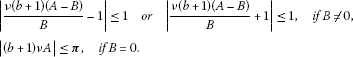If$f\in A\left(p\right)$with${J}_{p,s,b}\left(f\right)\left(z\right)\ne 0$for all$z\in {U}^{\ast }=U\mathrm{╲}\left\{0\right\}$, then

$\frac{{J}_{p,s-1,b}\left(f\right)\left(z\right)}{{J}_{p,s,b}\left(f\right)\left(z\right)}\prec \frac{1+Az}{1+Bz},$

implies

${\left(\frac{{J}_{p,s,b}\left(f\right)\left(z\right)}{{z}^{p}}\right)}^{\nu }\prec {q}_{1}\left(z\right),$

where

${q}_{1}\left(z\right)=\left\{\begin{array}{cc}{\left(1+Bz\right)}^{\nu \left(b+1\right)\left(A-B\right)/B},\hfill & \text{if}B\ne 0,\hfill \\ {e}^{\nu \left(b+1\right)Az},\hfill & \text{if}B=0,\hfill \end{array}$

is the best dominant.

Proof Let us put

$\phi \left(z\right)={\left(\frac{{J}_{p,s,b}\left(f\right)\left(z\right)}{{z}^{p}}\right)}^{\nu }\phantom{\rule{1em}{0ex}}\left(z\in U\right).$
(3.34)

Then φ is analytic in U, $\phi \left(0\right)=1$ and $\phi \left(z\right)\ne 0$ for all $z\in U$. Taking the logarithmic derivatives in both sides of (3.34) and using the identity (1.9), we have

$1+\frac{z{\phi }^{\mathrm{\prime }}\left(z\right)}{\nu \left(b+1\right)\phi \left(z\right)}=\frac{{J}_{p,s-1,b}\left(f\right)\left(z\right)}{{J}_{p,s,b}\left(f\right)\left(z\right)}\prec \frac{1+Az}{1+Bz}.$

Now the assertions of Theorem 8 follow by using Lemma 6 for $\gamma =\nu \left(b+1\right)$. □

Putting $B=-1$ and $A=1-2\sigma$, $0\le \sigma <1$, in Theorem 8, we obtain the following corollary.

Corollary 3 Assume that$\nu \in {\mathbb{C}}^{\ast }$satisfies either$|2\nu \left(b+1\right)\left(1-\sigma \right)-1|\le 1$or$|2\nu \left(b+1\right)\left(1-\sigma \right)+1|\le 1$. If$f\in A\left(p\right)$with${J}_{p,s,b}\left(f\right)\left(z\right)\ne 0$for$z\in {U}^{\ast }$, then

$Re\left\{\frac{{J}_{p,s-1,b}\left(f\right)\left(z\right)}{{J}_{p,s,b}\left(f\right)\left(z\right)}\right\}>\sigma \phantom{\rule{1em}{0ex}}\left(z\in U\right),$

implies

${\left(\frac{{J}_{p,s,b}\left(f\right)\left(z\right)}{{z}^{p}}\right)}^{\nu }\prec {q}_{2}\left(z\right)={\left(1-z\right)}^{-2\nu \left(b+1\right)\left(1-\sigma \right)},$

and${q}_{2}$is the best dominant.

Remark 2 Specializing the parameters s and b in the above results of this paper, we obtain the results for the corresponding operators ${F}_{\mu ,p}$, ${I}_{p}^{\alpha }$, ${J}_{p}^{\gamma }$ and ${J}_{p}^{\gamma }\left(l\right)$ which are defined in the introduction.

## References

1. Alexander JW: Functions which map the interior of the unit circle upon simple regions. Ann. Math., Ser. 2 1915, 17: 12–22. 10.2307/2007212

2. Choi J, Srivastava HM: Certain families of series associated with the Hurwitz-Lerch Zeta function. Appl. Math. Comput. 2005, 170: 399–409. 10.1016/j.amc.2004.12.004

3. Choi JH, Saigo M, Srivastava HM: Some inclusion properties of a certain family of integral operators. J. Math. Anal. Appl. 2002, 276: 432–445. 10.1016/S0022-247X(02)00500-0

4. El-Ashwah RM, Aouf MK: Some properties of new integral operator. Acta Univ. Apulensis, Mat.-Inform. 2010, 24: 51–61.

5. Ferreira C, Lopez JL: Asymptotic expansions of the Hurwitz-Lerch Zeta function. J. Math. Anal. Appl. 2004, 298: 210–224. 10.1016/j.jmaa.2004.05.040

6. Jung IB, Kim YC, Srivastava HM: The Hardy space of analytic functions associated with certain one parameter families of integral operators. J. Math. Anal. Appl. 1993, 176: 138–147. 10.1006/jmaa.1993.1204

7. Libera RJ: Some classes of regular univalent functions. Proc. Am. Math. Soc. 1969, 16: 755–758.

8. Lin S-D, Srivastava HM: Some families of the Hurwitz-Lerch Zeta functions and associated fractional derivative and other integral representations. Appl. Math. Comput. 2004, 154: 725–733. 10.1016/S0096-3003(03)00746-X

9. Lin S-D, Srivastava HM, Wang P-Y: Some expansion formulas for a class of generalized Hurwitz-Lerch Zeta functions. Integral Transforms Spec. Funct. 2006, 17: 817–827. 10.1080/10652460600926923

10. Liu J-L: Subordinations for certain multivalent analytic functions associated with the generalized Srivastava-Attiya operator. Integral Transforms Spec. Funct. 2007, 18: 207–216. 10.1080/10652460701208577

11. Luo Q-M, Srivastava HM: Some generalizations of the Apostol-Bernoulli and Apostol-Euler polynomials. J. Math. Anal. Appl. 2005, 308: 290–302. 10.1016/j.jmaa.2005.01.020

12. MacGregor TH: Radius of univalence of certain analytic functions. Proc. Am. Math. Soc. 1963, 14: 514–520. 10.1090/S0002-9939-1963-0148891-3

13. Miller SS, Mocanu PT: Differential subordinations and univalent functions. Mich. Math. J. 1981, 28: 157–171.

14. Miller SS, Mocanu PT: Differential Subordination. Theory and Applications. Marcel Dekker, New York; 2000.

15. Nehari PT: Conformal Mapping. McGraw-Hill, New York; 1952.

16. Obradovic M, Owa S: On certain properties for some classes of starlike functions. J. Math. Anal. Appl. 1990, 145: 357–364. 10.1016/0022-247X(90)90405-5

17. Pashkouleva DZ: The starlikeness and spiral-convexity of certain subclasses of analytic functions. In Current Topics in Analytic Function Theory. Edited by: Srivastava HM, Owa S. World Scientific, Singapore; 1992:266–273.

18. Shams S, Kulkarni SR, Jahangiri JM: Subordination properties of p -valent functions defined by integral operators. Int. J. Math. Math. Sci. 2006., 2006:

19. Singh R, Singh S: Convolution properties of a class of starlike functions. Proc. Am. Math. Soc. 1989, 106: 145–152. 10.1090/S0002-9939-1989-0994388-6

20. Srivastava HM, Attiya AA: An integral operator associated with the Hurwitz-Lerch Zeta function and differential subordination. Integral Transforms Spec. Funct. 2007, 18: 207–216. 10.1080/10652460701208577

21. Srivastava HM, Choi J: Series Associated with the Zeta and Related Functions. Kluwer Academic, Dordrecht; 2001.

22. Stankiewicz J, Stankiewicz Z: Some applications of Hadamard convolution in the theory of functions. Ann. Univ. Mariae Curie-Skl̄odowska, Sect. A 1986, 40: 251–265.

23. Whittaker ET, Watson GN: A Course of Modern Analysis: An Introduction to the General Theory of Infinite Processes and of Analytic Functions; with an Account of the Principal Transcendental Functions. Cambridge University Press, Cambridge; 1927.

24. Wilken DR, Feng J: A remark on convex and starlike functions. J. Lond. Math. Soc., Ser. 2 1980, 21: 287–290. 10.1112/jlms/s2-21.2.287

## Acknowledgements

The authors would like to thank the referees of the paper for their helpful suggestions.

## Author information

Authors

### Competing interests

The authors declare that they have no competing interests.

### Authors’ contributions

All authors read and approved the final manuscript.

## Rights and permissions

Reprints and Permissions

Aouf, M., Mostafa, A., Shahin, A. et al. Applications of differential subordinations for certain classes of p-valent functions associated with generalized Srivastava-Attiya operator. J Inequal Appl 2012, 153 (2012). https://doi.org/10.1186/1029-242X-2012-153